• 外极线
千次阅读
2018-03-26 13:47:55

两张视角相似的图片，通过提取特征点、描述子，然后进行匹配，再通过单应约束优化之后得到的匹配关系基本就是正确的匹配了。然后在此基础上想画出外极线，来验证一下匹配的结果是否正确。于是就写了一个简单的函数。假设已经得到了匹配的特征点了，下面的函数将会画出这些点对应的外极线。

[cpp]  view plain
1. //画出外极线,对应点已经经过了RANSAC优化
2. void DrawEpiLines(const Mat& img_1, const Mat& img_2, vector<Point2f>points1, vector<Point2f>points2){
3.
4.     cv::Mat F = cv::findFundamentalMat(points1, points2, CV_FM_8POINT);
5.         //首先根据对应点计算出两视图的基础矩阵，基础矩阵包含了两个相机的外参数关系
6.
7.        std::vector<cv::Vec<float, 3>> epilines1, epilines2;
8.     cv::computeCorrespondEpilines(points1, 1, F, epilines1);//计算对应点的外极线epilines是一个三元组(a,b,c)，表示点在另一视图中对应的外极线ax+by+c=0;
9.         cv::computeCorrespondEpilines(points2, 2, F, epilines2);
10.     //将图片转换为RGB图，画图的时候外极线用彩色绘制
11.     cv::Mat img1, img2;
12.     if (img_1.type() == CV_8UC3)
13.     {
14.          img_1.copyTo(img1);
15.          img_2.copyTo(img2);
16.      }
17.      else if (img_1.type() == CV_8UC1)
18.      {
19.          cvtColor(img_1, img1, COLOR_GRAY2BGR);
20.          cvtColor(img_2, img2, COLOR_GRAY2BGR);
21.      }
22.      else
23.      {
24.          cout << "unknow img type\n" << endl;
25.          exit(0);
26.      }
27.
28.      cv::RNG& rng = theRNG();
29.      for (uint i = 0; i < points2.size(); i++)
30.     {
31.         Scalar color = Scalar(rng(256), rng(256), rng(256));//随机产生颜色
32.
33.         circle(img2, points2[i], 5, color);//在视图2中把关键点用圆圈画出来，然后再绘制在对应点处的外极线
34.         line(img2, Point(0, -epilines1[i] / epilines1[i]), Point(img2.cols, -(epilines1[i] + epilines1[i] * img2.cols) / epilines1[i]), color);
35.                 //绘制外极线的时候，选择两个点，一个是x=0处的点，一个是x为图片宽度处
36.          circle(img1, points1[i], 4, color);
37.          line(img1, cv::Point(0, -epilines2[i] / epilines2[i]), cv::Point(img1.cols, -(epilines2[i] + epilines2[i] * img1.cols) / epilines2[i]), color);
38.
39.     }
40.     cv::imshow("img2 epiline1", img2);
41.     cv::imshow("img1 epiline2", img1);
42.
43.     waitKey(0);
44. }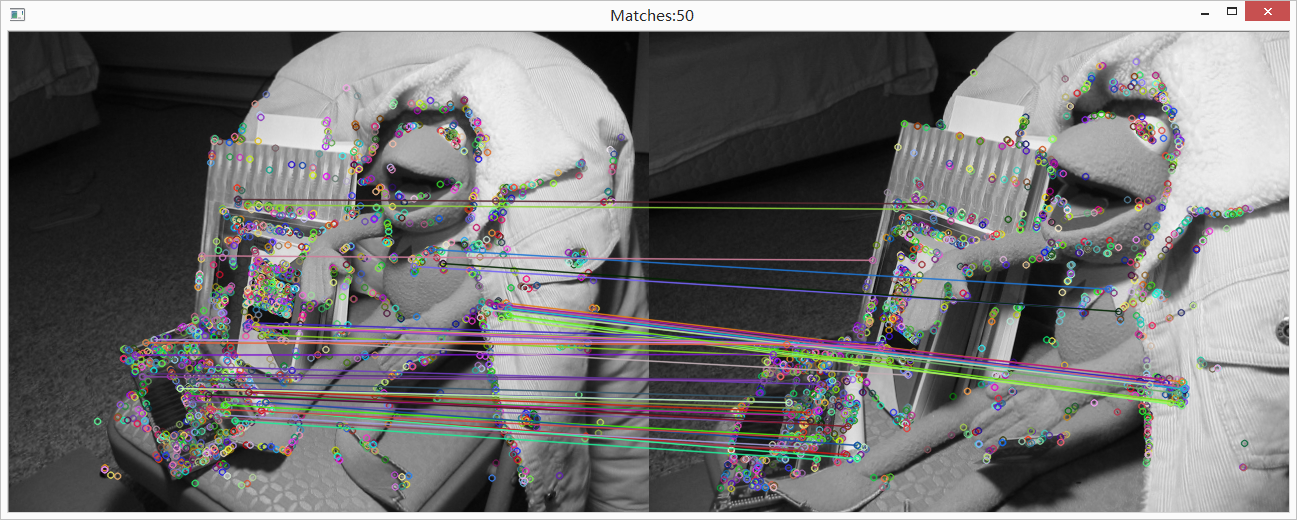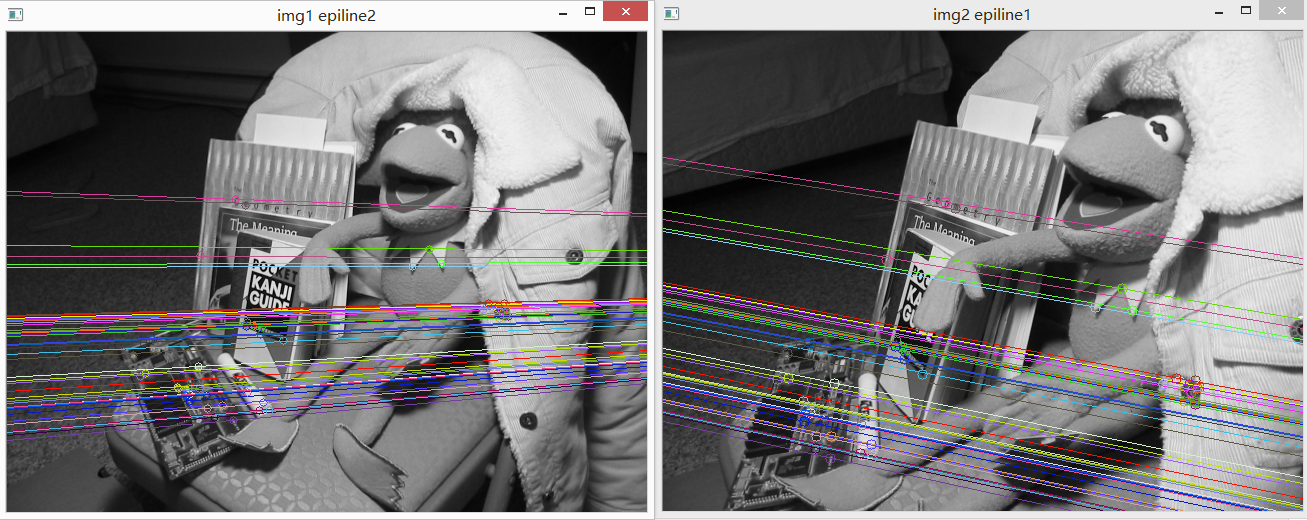更多相关内容
• 三目立体视觉外极线校正及其 DEFG实现方法安路平H 贾云得H 李鸣翔@北京理工大学 信息科学技术学院计算机科学工程系H北京要<提出一种三目立体视觉外极线校正的
• 提出一种三目立体视觉外极线校正的快速算法，3个摄像机呈直角三角形配置，且已知摄像机透视投影矩阵。根据校正基本约束确定校正后的图像平面，利用图像不变形约束计算校正所需的三阶矩阵，用于在平面仿射坐标系下对...
• 详细而全面地介绍摄像机模型和外极线几何的相关知识，针孔模型，透视投影，仿射摄像机等
• 针对三维运动捕捉系统标记点匹配中存在标记点交叠等情况和速度要求，提出利用平行相机结构，使用统计匹配像素点的平均高度差计算相机间的高度差，并将其与外极线约束相结合将匹配标记点搜索范围限制在一个特定区域内...
• 使用外极线作为第二个输入，通过在外极线上对每个特征点寻找最佳的匹配来找到更多的匹配。 from PIL import Image from numpy import * from pylab import * import numpy as np from PCV.geometry import camera ...

# 一、原理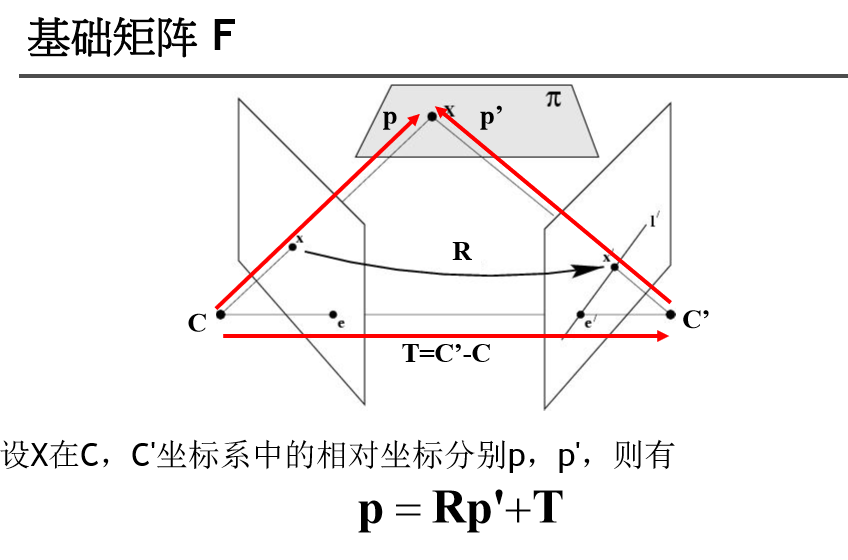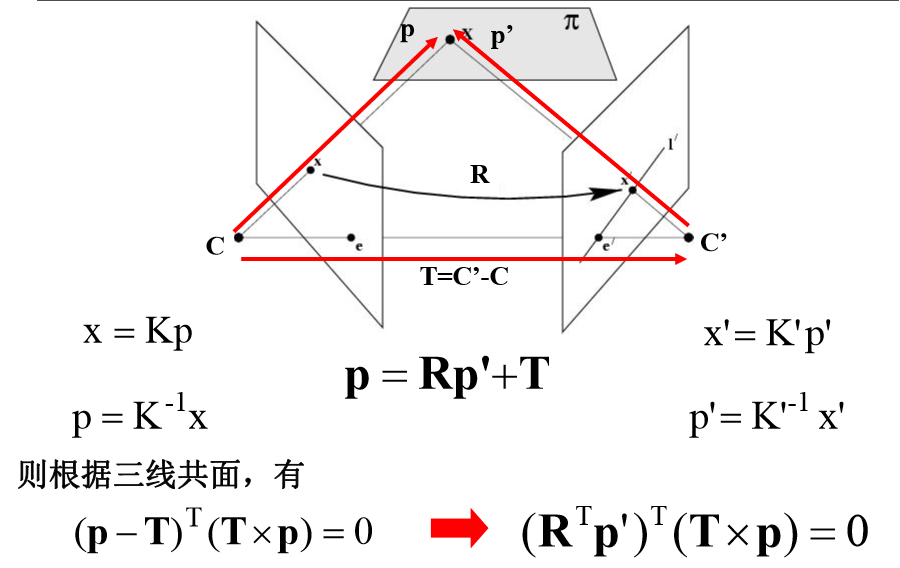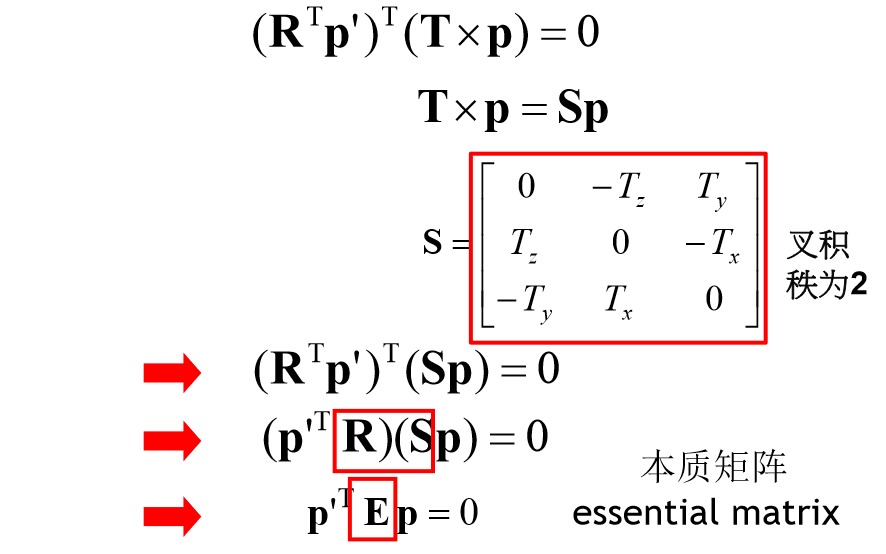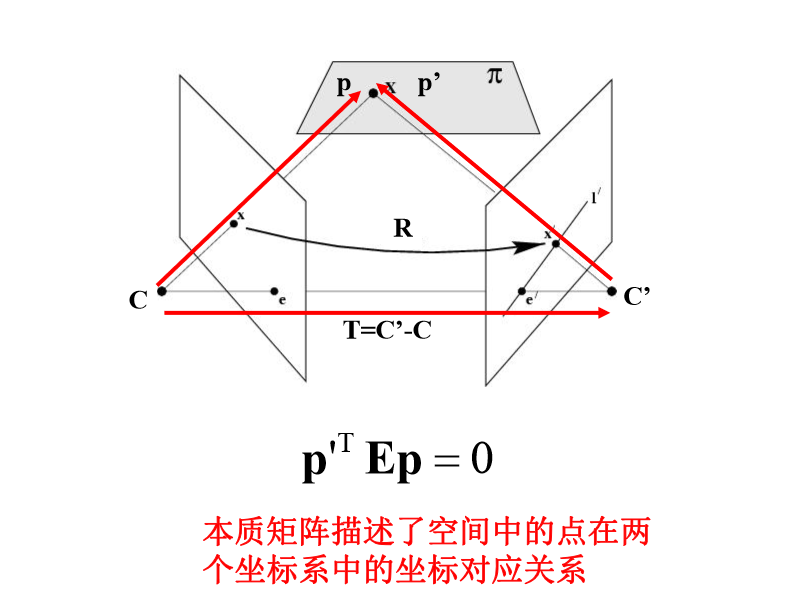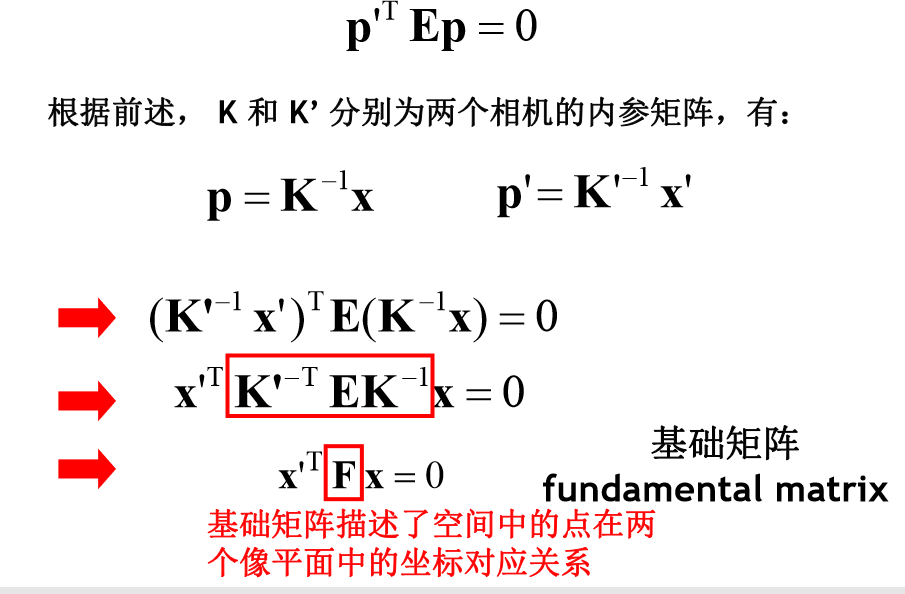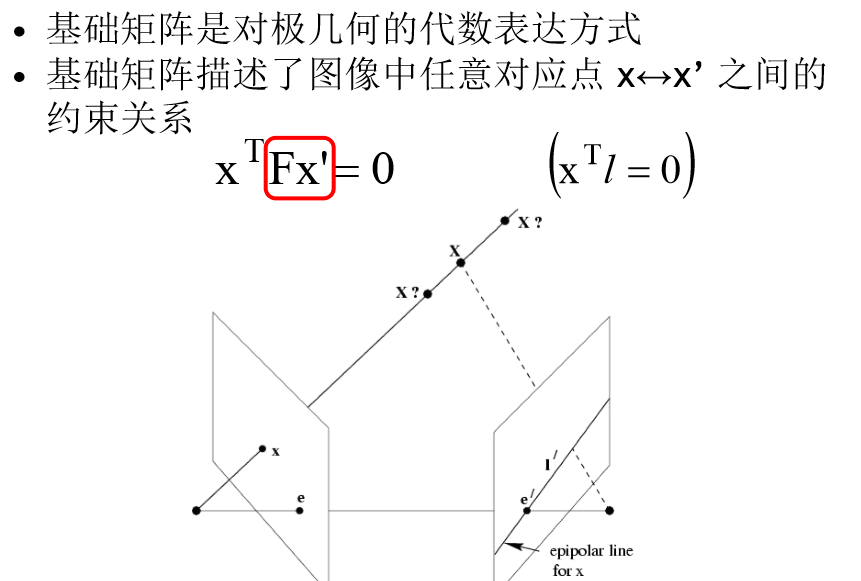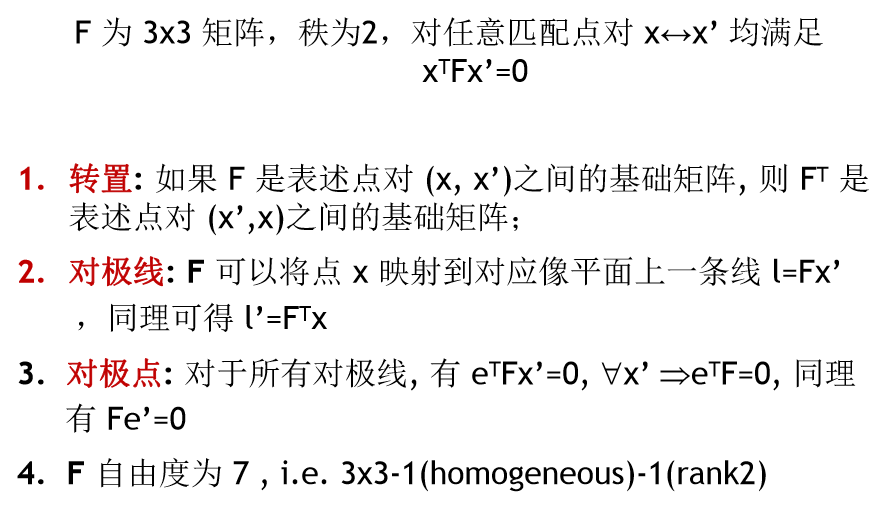# 二、代码

功能：计算图像对的特征匹配，并估计基础矩阵。使用外极线作为第二个输入，通过在外极线上对每个特征点寻找最佳的匹配来找到更多的匹配。

from PIL import Image
from numpy import *
from pylab import *
import numpy as np

from PCV.geometry import camera
from PCV.geometry import homography
from PCV.geometry import sfm
from PCV.localdescriptors import sift

im1 = array(Image.open('images4/1.jpg'))
sift.process_image('images4/1.jpg', 'im1.sift')

im2 = array(Image.open('images4/2.jpg'))
sift.process_image('images4/2.jpg', 'im2.sift')

matches = sift.match_twosided(d1, d2)
ndx = matches.nonzero()

x1 = homography.make_homog(l1[ndx, :2].T)
ndx2 = [int(matches[i]) for i in ndx]
x2 = homography.make_homog(l2[ndx2, :2].T)

d1n = d1[ndx]
d2n = d2[ndx2]
x1n = x1.copy()
x2n = x2.copy()

figure(figsize=(16,16))
sift.plot_matches(im1, im2, l1, l2, matches, True)
show()

#def F_from_ransac(x1, x2, model, maxiter=5000, match_threshold=1e-6):
def F_from_ransac(x1, x2, model, maxiter=5000, match_threshold=1e-6):
""" Robust estimation of a fundamental matrix F from point
correspondences using RANSAC (ransac.py from
http://www.scipy.org/Cookbook/RANSAC).

input: x1, x2 (3*n arrays) points in hom. coordinates. """

from PCV.tools import ransac
data = np.vstack((x1, x2))
d = 10 # 20 is the original
# compute F and return with inlier index
F, ransac_data = ransac.ransac(data.T, model,
8, maxiter, match_threshold, d, return_all=True)
return F, ransac_data['inliers']

# find F through RANSA43C
model = sfm.RansacModel()
F, inliers = F_from_ransac(x1n, x2n, model, maxiter=5000, match_threshold=1e-3)
print (F)

P1 = array([[1, 0, 0, 0], [0, 1, 0, 0], [0, 0, 1, 0]])
P2 = sfm.compute_P_from_fundamental(F)#Computes the second camera matrix

print (P2)
print (F)

# triangulate inliers and remove points not in front of both cameras
X = sfm.triangulate(x1n[:, inliers], x2n[:, inliers], P1, P2)

# plot the projection of X
cam1 = camera.Camera(P1)
cam2 = camera.Camera(P2)
x1p = cam1.project(X)
x2p = cam2.project(X)

figure(figsize=(16, 16))
imj = sift.appendimages(im1, im2)
imj = vstack((imj, imj))

imshow(imj)

cols1 = im1.shape
rows1 = im1.shape
for i in range(len(x1p)):
if (0<= x1p[i]<cols1) and (0<= x2p[i]<cols1) and (0<=x1p[i]<rows1) and (0<=x2p[i]<rows1):
plot([x1p[i], x2p[i]+cols1],[x1p[i], x2p[i]],'c')
axis('off')
show()

d1p = d1n[inliers]
d2p = d2n[inliers]

im3 = array(Image.open('images4/3.jpg'))
sift.process_image('images4/3.jpg', 'im3.sift')

matches13 = sift.match_twosided(d1p, d3)

ndx_13 = matches13.nonzero()
x1_13 = homography.make_homog(x1p[:, ndx_13])
ndx2_13 = [int(matches13[i]) for i in ndx_13]
x3_13 = homography.make_homog(l3[ndx2_13, :2].T)

figure(figsize=(16, 16))
imj = sift.appendimages(im1, im3)
imj = vstack((imj, imj))#vstack函数功能：垂直（按照行顺序）地把数组给堆叠起来

imshow(imj)

cols1 = im1.shape
rows1 = im1.shape
for i in range(len(x1_13)):
if (0<= x1_13[i]<cols1) and (0<= x3_13[i]<cols1) and (0<=x1_13[i]<rows1) and (0<=x3_13[i]<rows1):
plot([x1_13[i], x3_13[i]+cols1],[x1_13[i], x3_13[i]],'c')
axis('off')
show()

P3 = sfm.compute_P(x3_13, X[:, ndx_13])

print (P3)

print (P1)
print (P2)
print (P3)


# 三、实验结果及分析

## 1.室内图像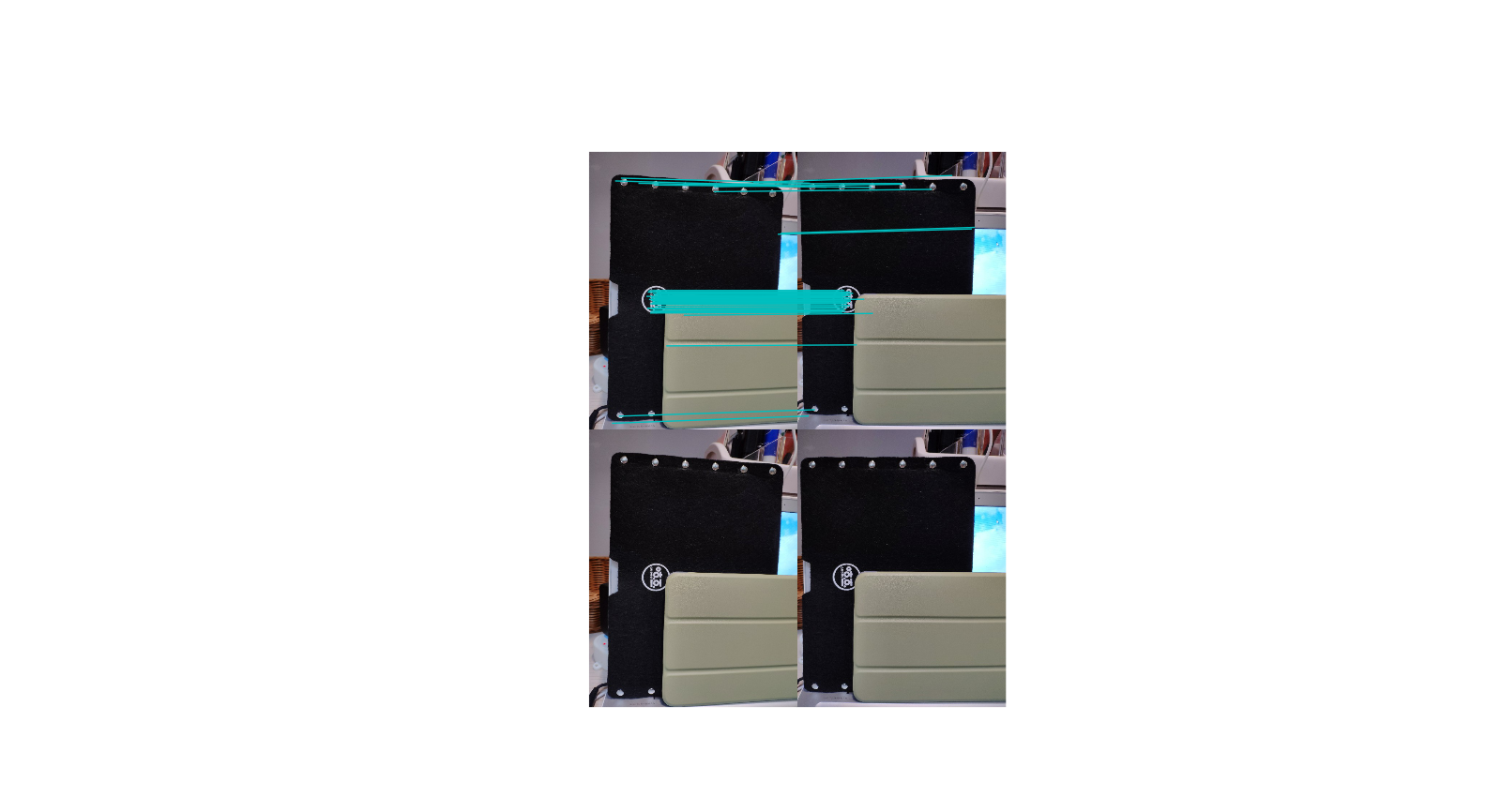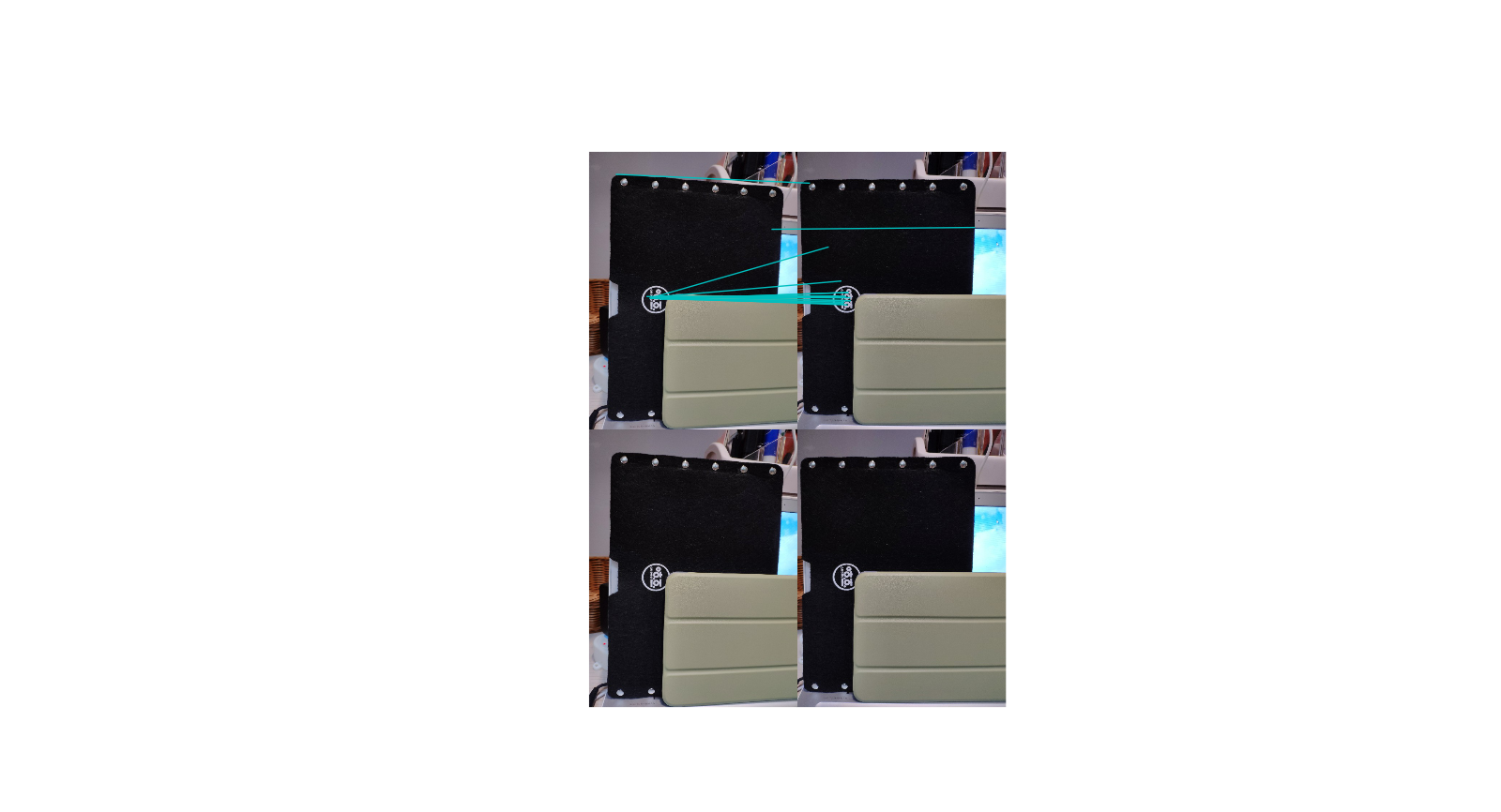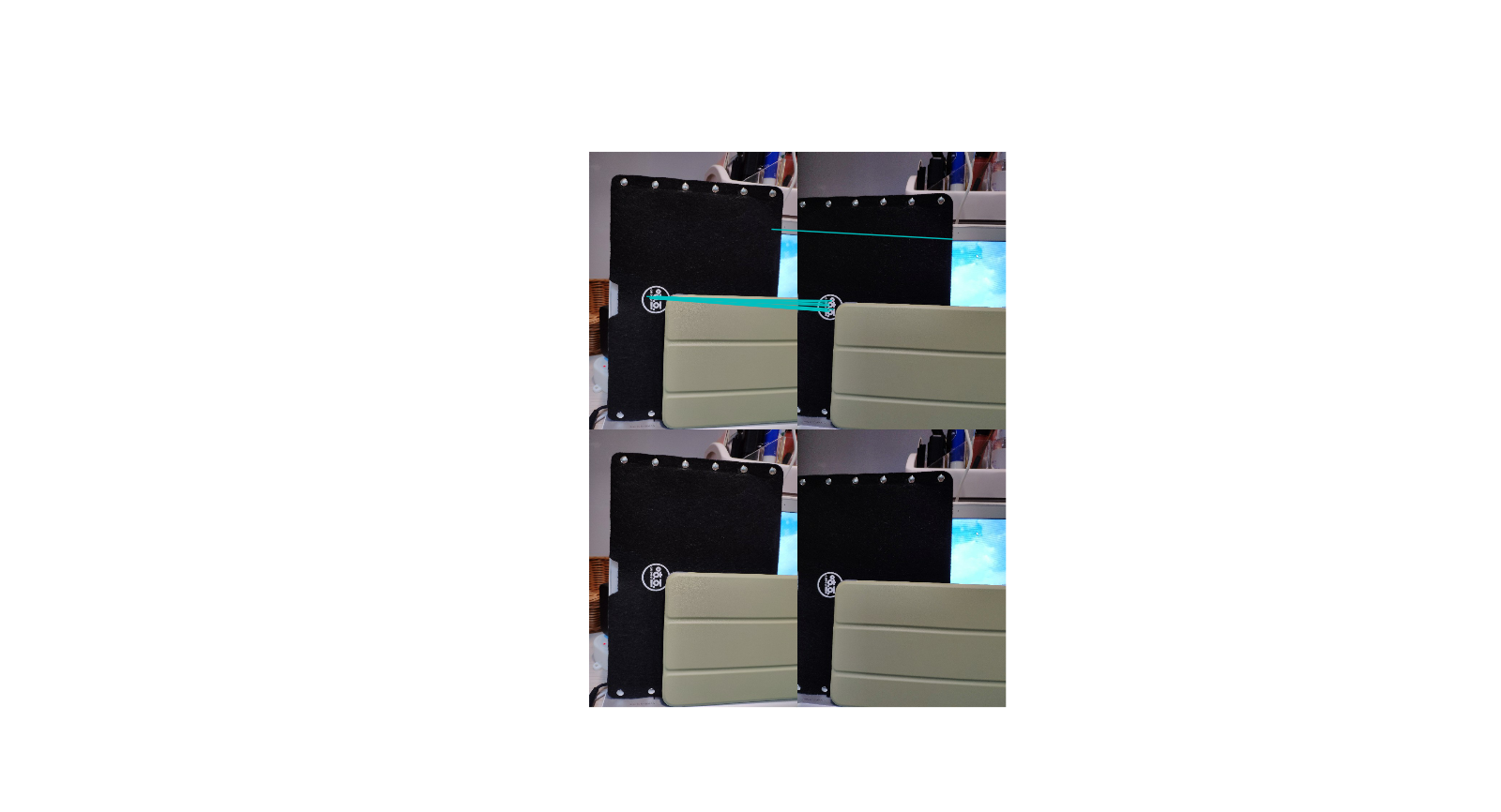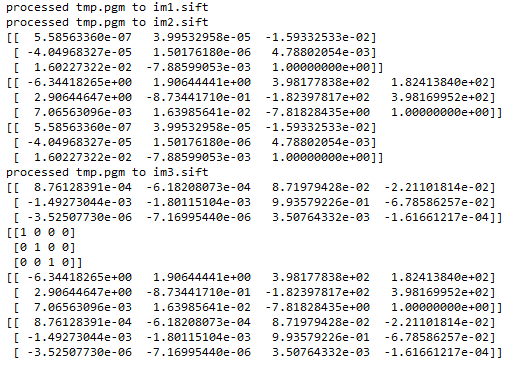## 2.室外远景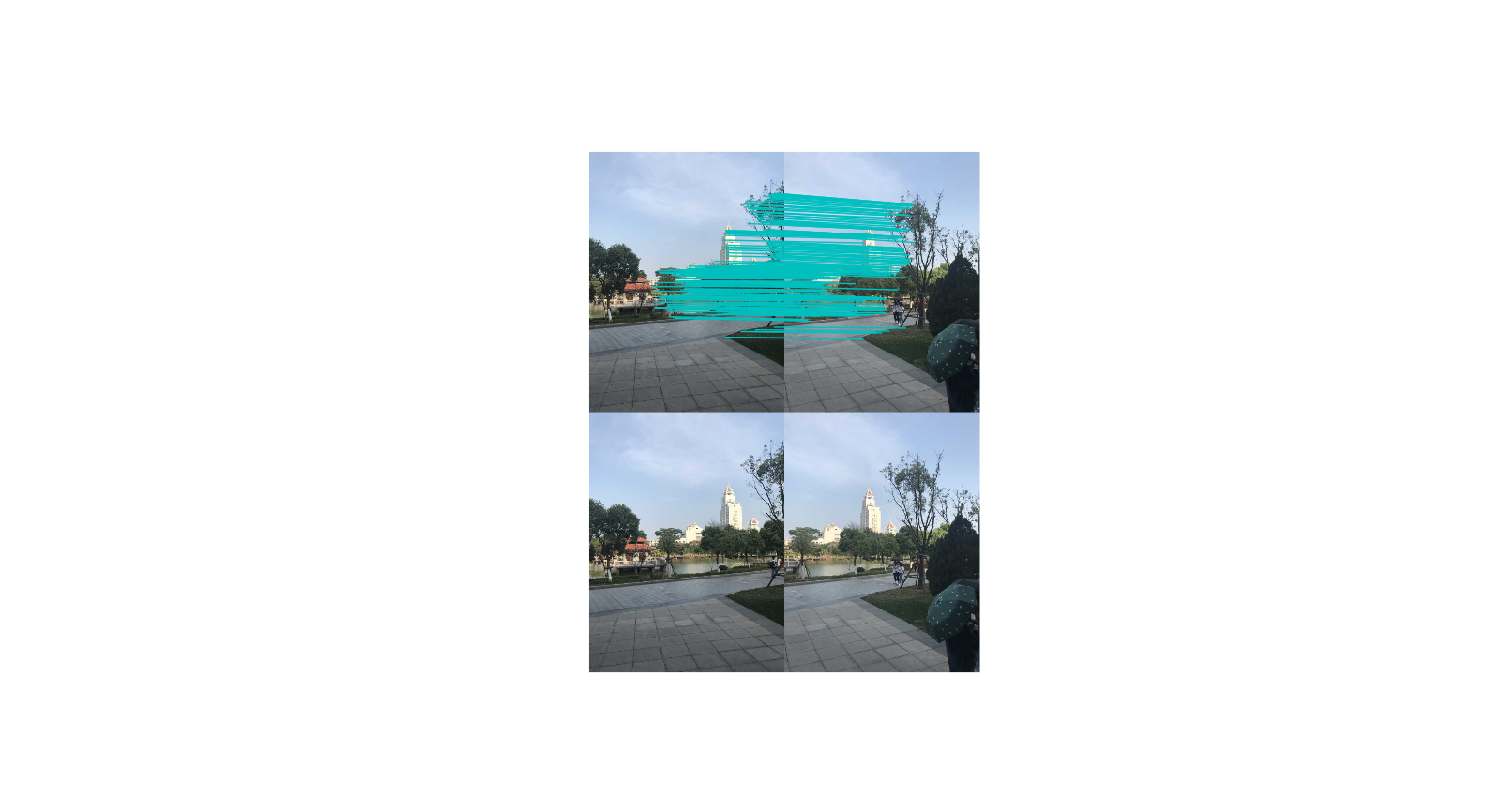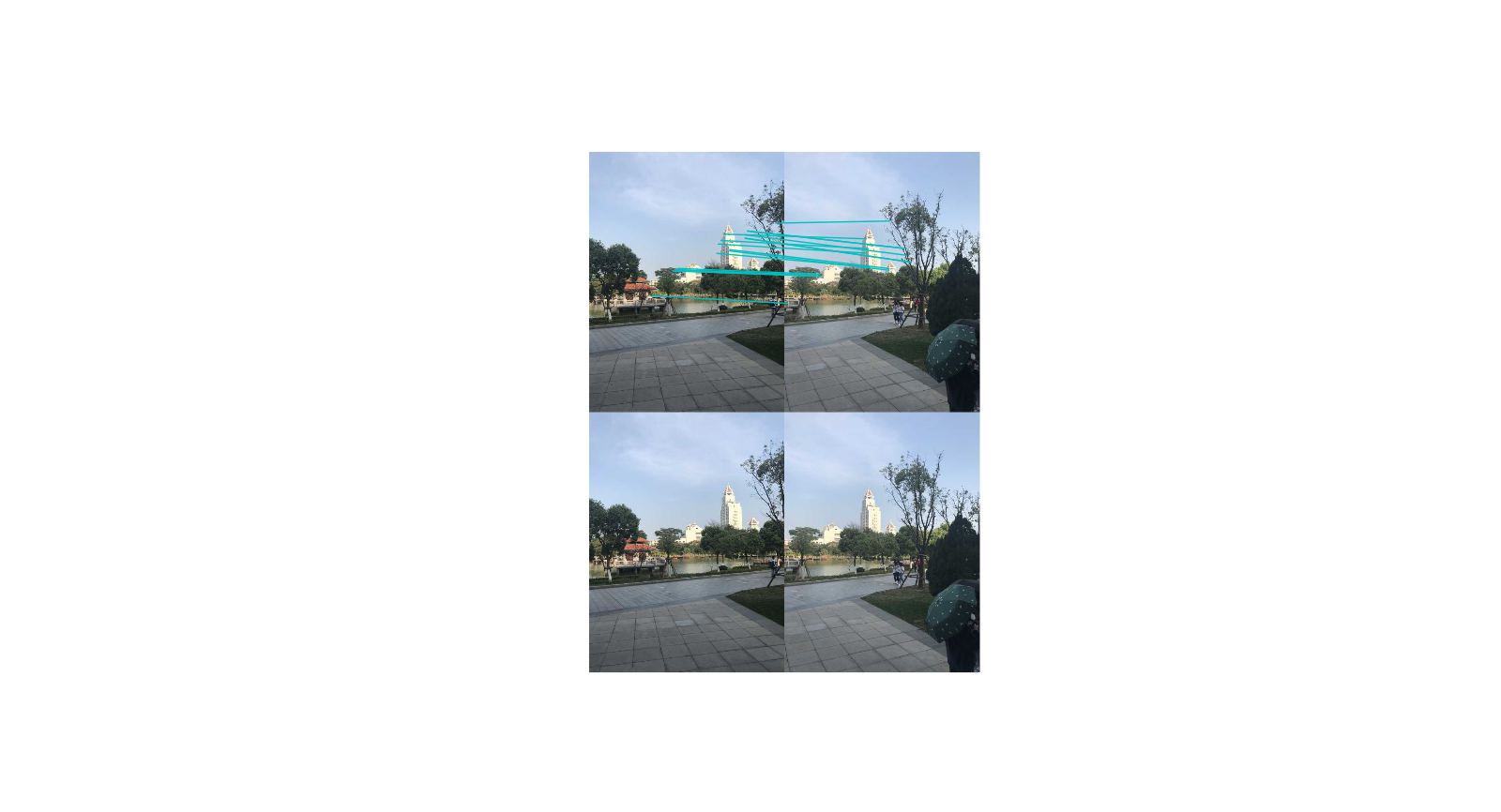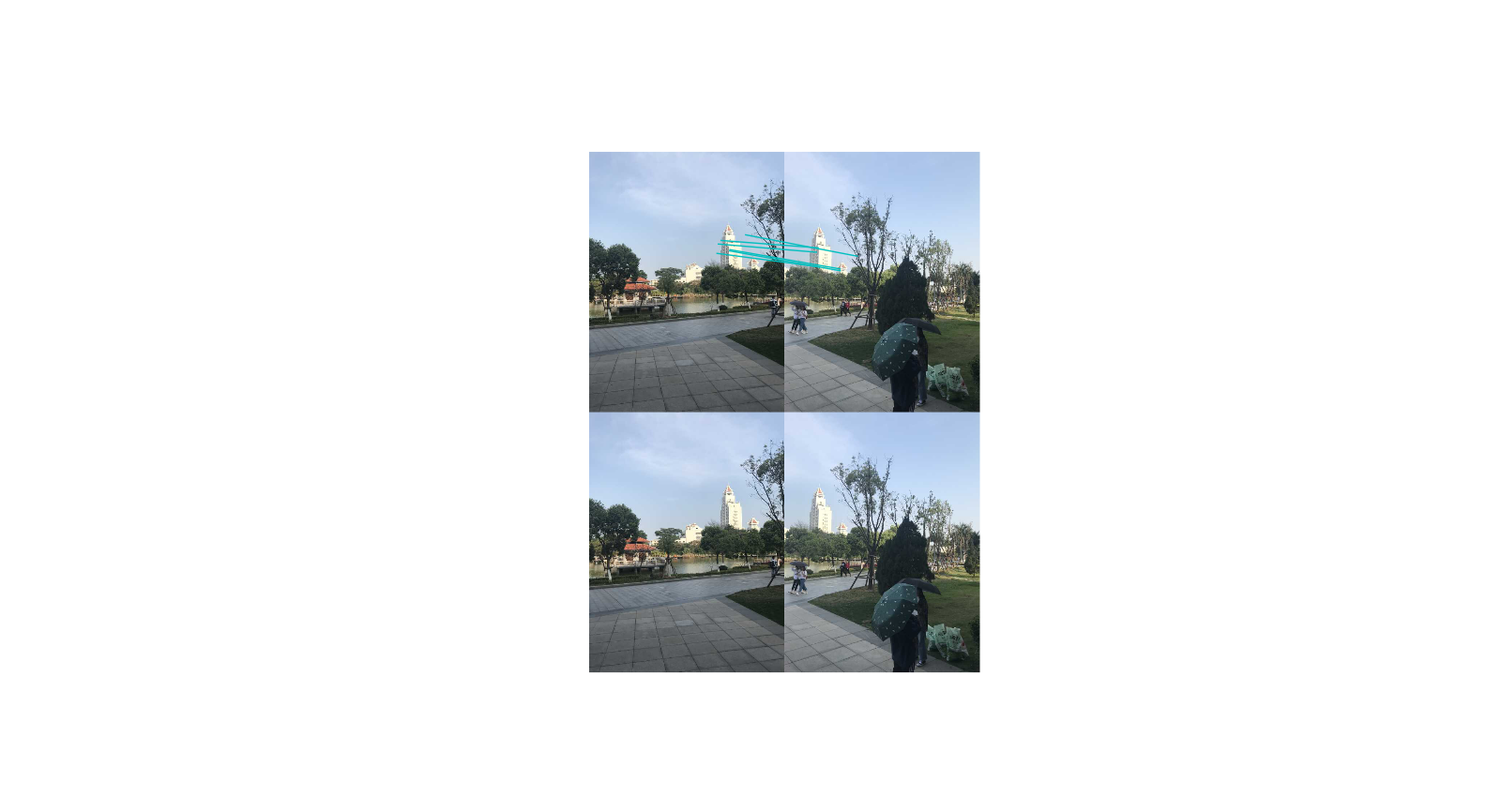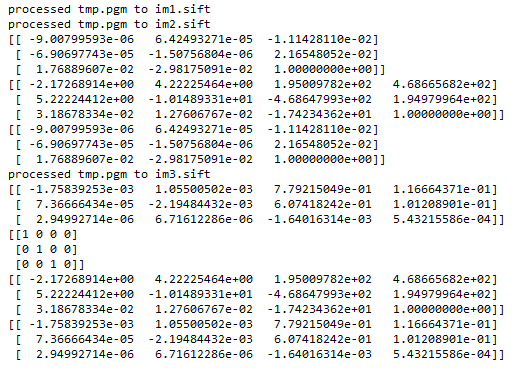## 3.室外近景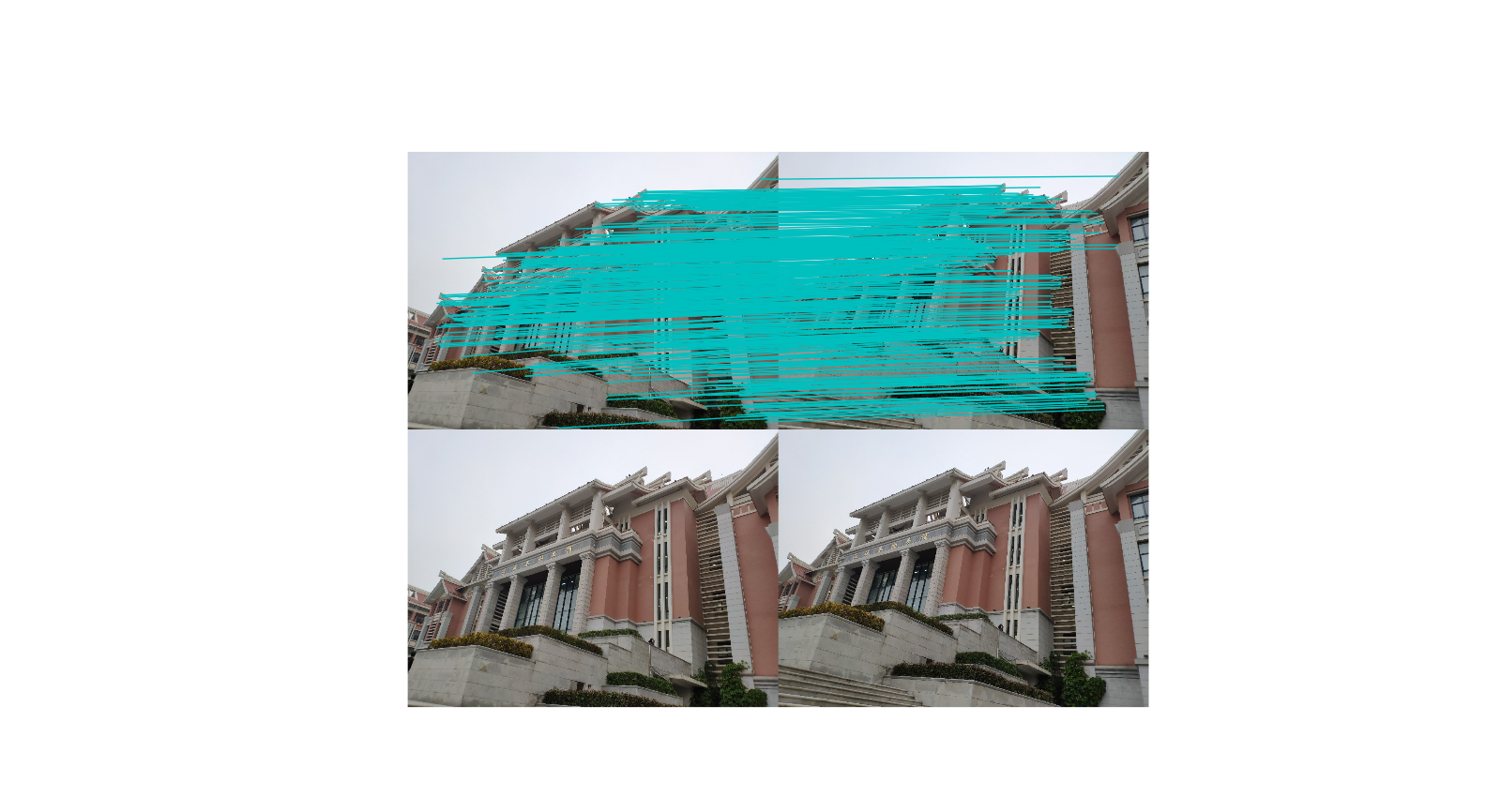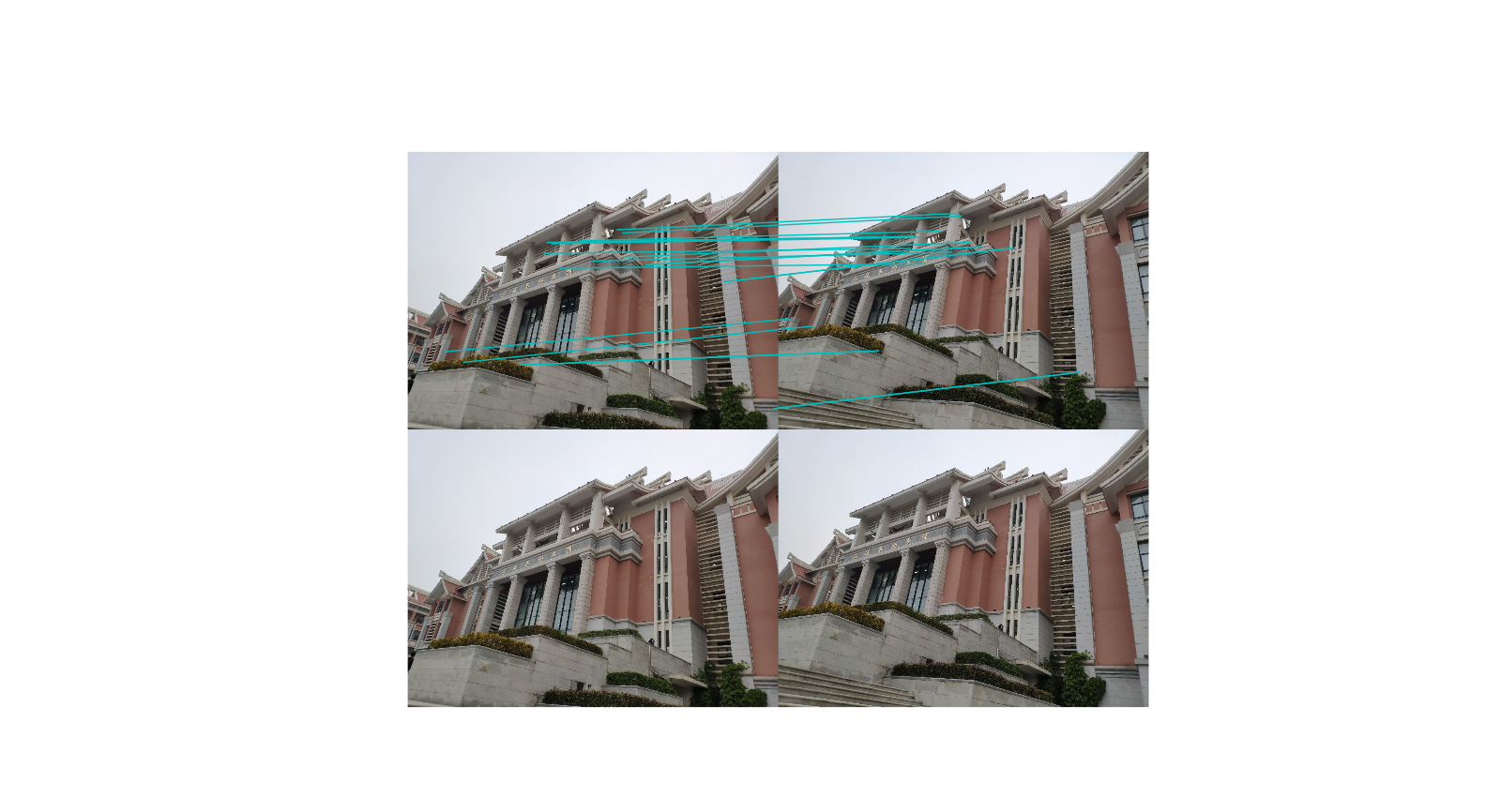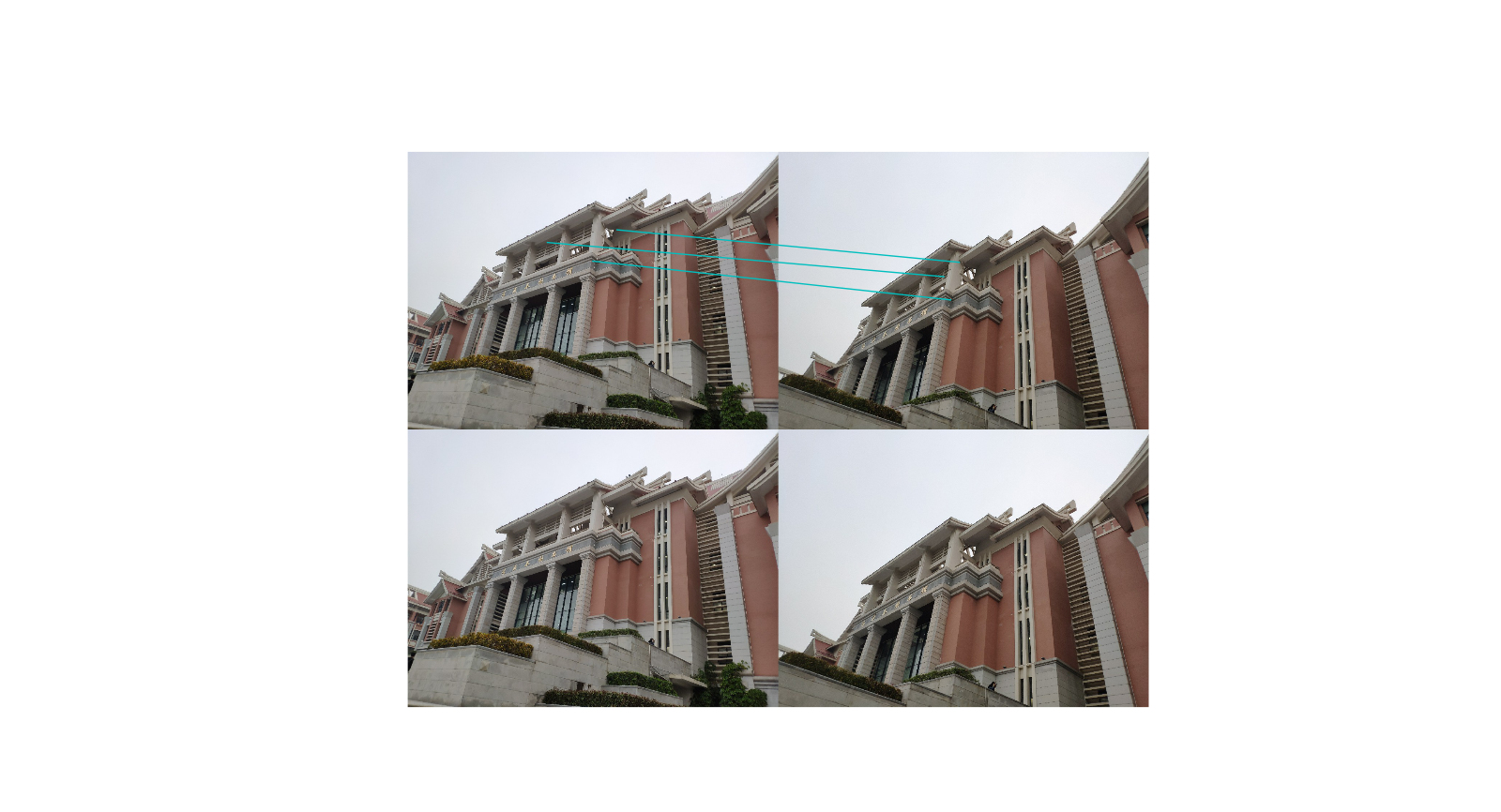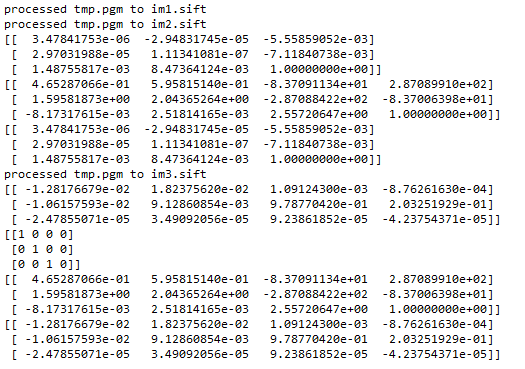## 2.分析

近景匹配效果较好，远景易出现错配，算法还不够精确。

展开全文• 融合深度学习与外极线约束的三维人体位姿测量方法.pdf
• 模式识别基础，在掌握摄像机基础模型后，运用到机器视觉和图像处理中的详细推导和公式变换。
• 本文提出一种基于极线约束的最小二乘图像匹配方法。该方法利用两幅图像间的极线约束得到多个预匹配点，确定预匹配区域，然后用用最小二乘法获得最佳匹配
• 1.1 极几何 极几何是研究两幅图像之间存在的几何。它和场景结构无关，只依赖于摄像机的内外参数。研究这种几何可以用在图像匹配、三维重建方面。 如上图所示，C1C_1C1​和C2C_2C2​是两个摄像头的光心，X为空间...

## 一、实验原理

#### 1.1 外极几何

外极几何是研究两幅图像之间存在的几何。它和场景结构无关，只依赖于摄像机的内外参数。研究这种几何可以用在图像匹配、三维重建方面。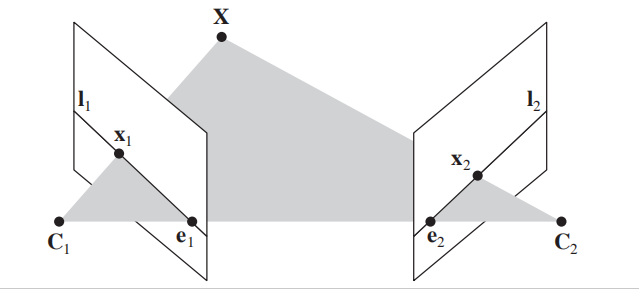如上图所示， C 1 C_1 C 2 C_2 是两个摄像头的光心，X为空间中的一点， x 1 x_1 x 2 x_2 是点X在两个摄像头成的像中的投影。

基本概念：
基线： 连接两个摄像机光点 C 1 C_1 C 2 C_2 的直线。
外极点： 基线与图像平面的交点 e 1 e_1 e 2 e_2
外极平面: 过基线的平面 C 1 C_1 C 2 C_2 X
外极线： 对极平面与图像平面的交线 l 1 l_1 l 2 l_2
外极线约束： 假设 x 1 x_1 x 2 x_2 分别是空间中同一点在两个不同视平面上的像点，则 x 1 x_1 一定在 l 1 l_1 上， x 2 x_2 一定在 l 2 l_2
如果已经知道相机的参数，那么在重建过程中遇到的问题就是两幅图像之间的关系，外极线约束的主要作用就是限制对应特征点的搜索范围，将对应特征点的搜索限制在极线上。

#### 1.2 基础矩阵

在计算机视觉中，基础矩阵（Fundamental matrix）F是一个3×3的矩阵，表达了立体像对的像点之间的对应关系。在对极几何中，对于立体像对中的一对同名点，它们的齐次化图像坐标分别为x与 x’， F x F_x 表示一条必定经过x’的直线（极线）。这意味着立体像对的所有同名点对都满足： x ′ T x'^T F x F_x =0

F矩阵中蕴含了立体像对的两幅图像在拍摄时相互之间的空间几何关系（外参数）以及相机检校参数（内参数），包括旋转、位移、像主点坐标和焦距。因为F矩阵的秩为2，并且可以自由缩放（尺度化），所以只需7对同名点即可估算出F的值。

基础矩阵性质
（1）基础矩阵是秩为2、自由度为7的齐次矩阵。
（2）若x与 x’是两幅图上的对应点，那么 x ′ T x'^T F x F_x =0
（3）l’是对应于x的对极线，l′= F x F_x
（4）若e是第二个摄像机光心在第一幅图像上的极点，那么Fe=0 。

#### 1.1 八点算法

八点法是通过对应点来计算基础矩阵的算法。

基本矩阵是由该方程定义的： x ′ T x'^T F x F_x =0

其中x↔x′是两幅图像的任意一对匹配点。由于每一组点的匹配提供了计算F系数的一个线性方程，当给定至少7个点（3×3的齐次矩阵减去一个尺度，以及一个秩为2的约束），方程就可以计算出未知的F。我们记点的坐标为 x = ( x , y , 1 ) T x=(x,y,1)^T x ′ = ( x ′ , y ′ , 1 ) T x′=(x′,y′,1)^T

又因为F为：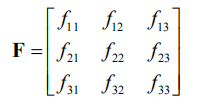所以可得到方程：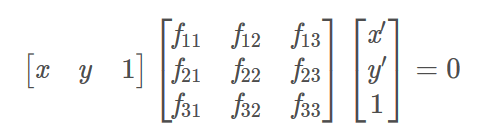即相应方程式为: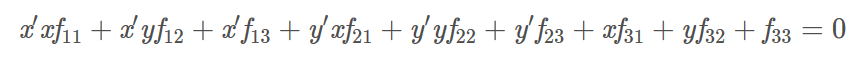给定nn组点的集合，我们有如下方程：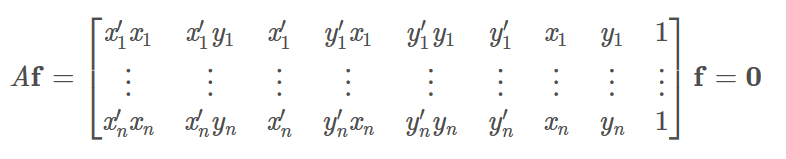如果存在确定（非零）解，则系数矩阵A的秩最多是8。由于F是齐次矩阵，所以如果矩阵A的秩为8，则在差一个尺度因子的情况下解是唯一的。可以直接用线性算法解得。

如果由于点坐标存在噪声则矩阵A的秩可能大于8（也就是等于9，由于A是n×9的矩阵）。这时候就需要求最小二乘解，这里就可以用SVD来求解，f的解就是系数矩阵A最小奇异值对应的奇异向量，也就是A奇异值分解后 A = U D V T A=UDV^T 中矩阵V的最后一列矢量，这是在解矢量f在约束 ||f|| 下取 ||Af|| 最小的解。以上算法是解基本矩阵的基本方法，称为8点算法。

上述求解后的F不一定能满足秩为2的约束，因此还要在F的基础上加以约束。通过SVD分解可以解决，令 F = U Σ V T F=UΣV^T ，则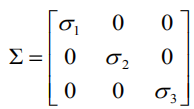因为要秩为2，所以取最后一个元素设置为0，则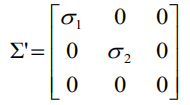最终的解为： F ′ = U Σ ′ V T F'=UΣ'V^T

## 二、代码

# coding: utf-8
from PIL import Image
from numpy import *
from pylab import *
import numpy as np
from PCV.geometry import homography, camera, sfm
from PCV.localdescriptors import sift

# 提取特征
im1 = array(Image.open('E:/code/image/five/5.jpg'))
sift.process_image('E:/code/image/five/5.jpg', 'im1.sift')

im2 = array(Image.open('E:/code/image/five/6.jpg'))
sift.process_image('E:/code/image/five/6.jpg', 'im2.sift')

matches = sift.match_twosided(d1, d2)

ndx = matches.nonzero()
x1 = homography.make_homog(l1[ndx, :2].T)  # 将点集转化为齐次坐标表示
ndx2 = [int(matches[i]) for i in ndx]
x2 = homography.make_homog(l2[ndx2, :2].T)  # 将点集转化为齐次坐标表示

d1n = d1[ndx]
d2n = d2[ndx2]
x1n = x1.copy()
x2n = x2.copy()

figure(figsize=(16, 16))
sift.plot_matches(im1, im2, l1, l2, matches, True)  # 可视化
show()

import sfm111
## 计算 F
F = sfm111.compute_fundamental(x1n,x2n)
print(F)

def F_from_ransac(x1, x2, model, maxiter=5000, match_threshold=1e-6):
"""
使用RANSAC从点对应中稳健估计基本矩阵F.
（来自http://www.scipy.org/Cookbook/RANSAC的ransac.py）。
input: x1, x2 (3*n arrays) points in hom. coordinates. """

from PCV.tools import ransac
data = np.vstack((x1, x2))
d = 10  # 20 is the original
# 计算F并返回inlier索引
F, ransac_data = ransac.ransac(data.T, model,
7, maxiter, match_threshold, d, return_all=True)
return F, ransac_data['inliers']

# 通过RANSAC找到F.
model = sfm.RansacModel()
F, inliers = F_from_ransac(x1n, x2n, model, maxiter=5000, match_threshold=1e-3)

P1 = array([[1, 0, 0, 0], [0, 1, 0, 0], [0, 0, 1, 0]])
P2 = sfm.compute_P_from_fundamental(F)  # 计算第二个相机矩阵

# print P2
print 'F is'
print F

X = sfm.triangulate(x1n[:, inliers], x2n[:, inliers], P1, P2)

# 绘制X的投影
cam1 = camera.Camera(P1)
cam2 = camera.Camera(P2)
x1p = cam1.project(X)
x2p = cam2.project(X)

figure(figsize=(16, 16))
imj = sift.appendimages(im1, im2)
imj = vstack((imj, imj))

imshow(imj)

cols1 = im1.shape
rows1 = im1.shape

#for i in range(len(x1p)):
#    if (0 <= x1p[i] < cols1) and (0 <= x2p[i] < cols1) and (0 <= x1p[i] < rows1) and (0 <= x2p[i] < rows1):
#        plot([x1p[i], x2p[i] + cols1], [x1p[i], x2p[i]], 'c')
#
#for i in range(5):
#    plot([x1p[0,i],x2p[i],x1p[1,i],x2p[i]],'o')
#axis('off')
#show()

def compute_epipole(F):
""" 从基础矩阵 F 中计算右极点（可以使用 F.T 获得左极点）"""
# 返回 F 的零空间（Fx=0）
U,S,V = linalg.svd(F)
e = V[-1]
return e/e

def plot_epipolar_line(im,F,x,epipole=None,show_epipole=True):
""" 在图像中，绘制外极点和外极线 F×x=0。F 是基础矩阵，x 是另一幅图像中的点 """
m,n = im.shape[:2]
line = dot(F,x)
# 外极线参数和值
t = linspace(0,n,100)
lt = array([(line+line*tt)/(-line) for tt in t])
# 仅仅处理位于图像内部的点和线
ndx = (lt>=0) & (lt<m)
plot(t[ndx],lt[ndx],linewidth=2)
if show_epipole:
if epipole is None:
epipole = compute_epipole(F)
plot(epipole/epipole,epipole/epipole,'r*')

e = compute_epipole(F)
for i in range(len(x1p)):
plot_epipolar_line(im1,F,x2[:,i],e,False)
axis('off')
#figure()
#imshow(im2)
# 分别绘制每个点，这样会绘制出和线同样的颜色
for i in range(len(x1p)):
plot(x2[0,i],x2[1,i],'o')
axis('off')

d1p = d1n[inliers]
d2p = d2n[inliers]



## 三、实验内容及分析

#### 3.1求解图像之间的基础矩阵

##### 3.1.1 实验结果
1. 左右变换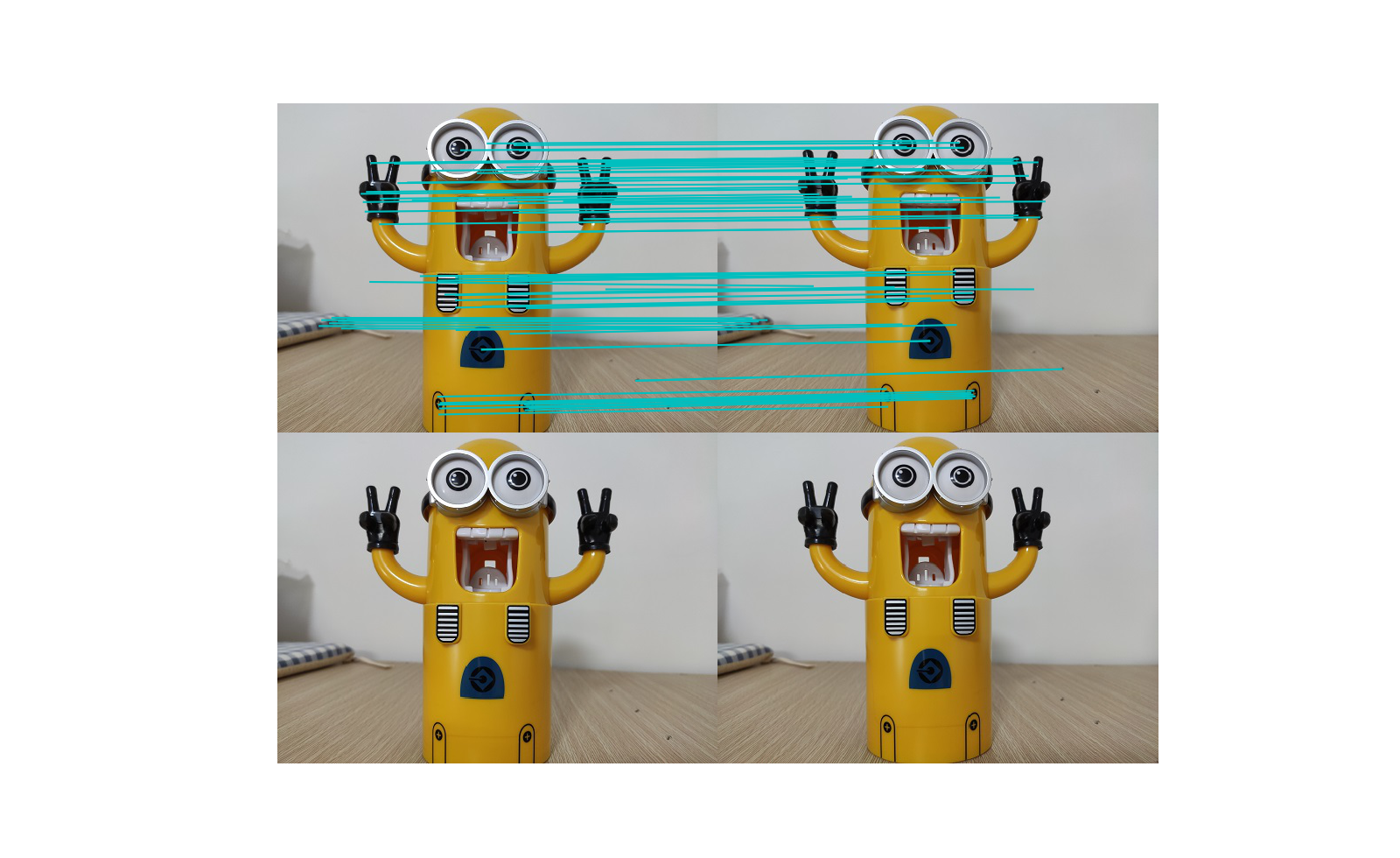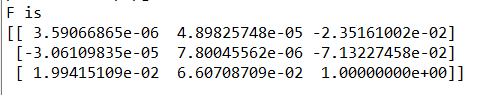2. 平移变换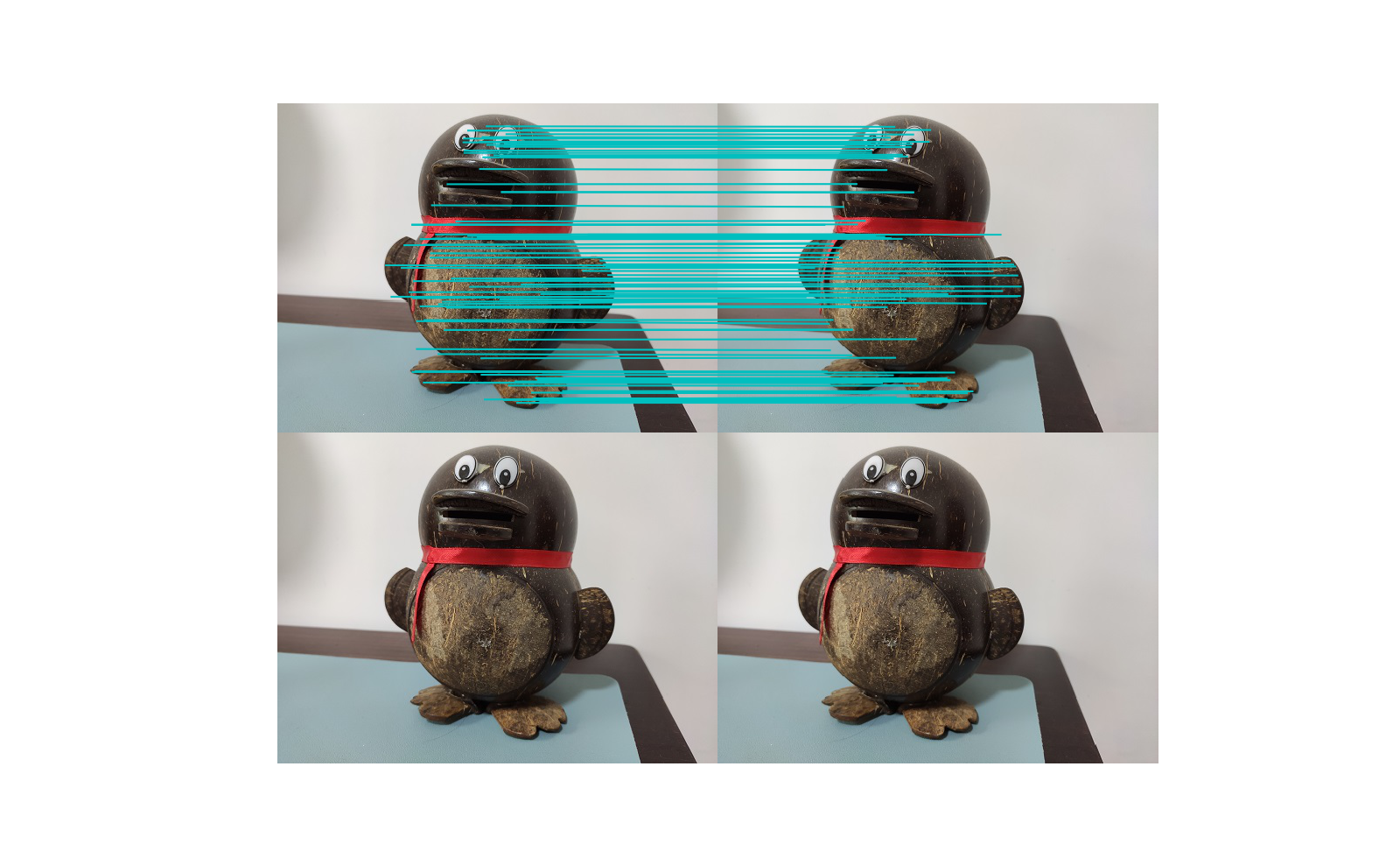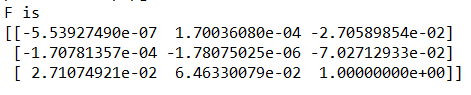3. 前后变换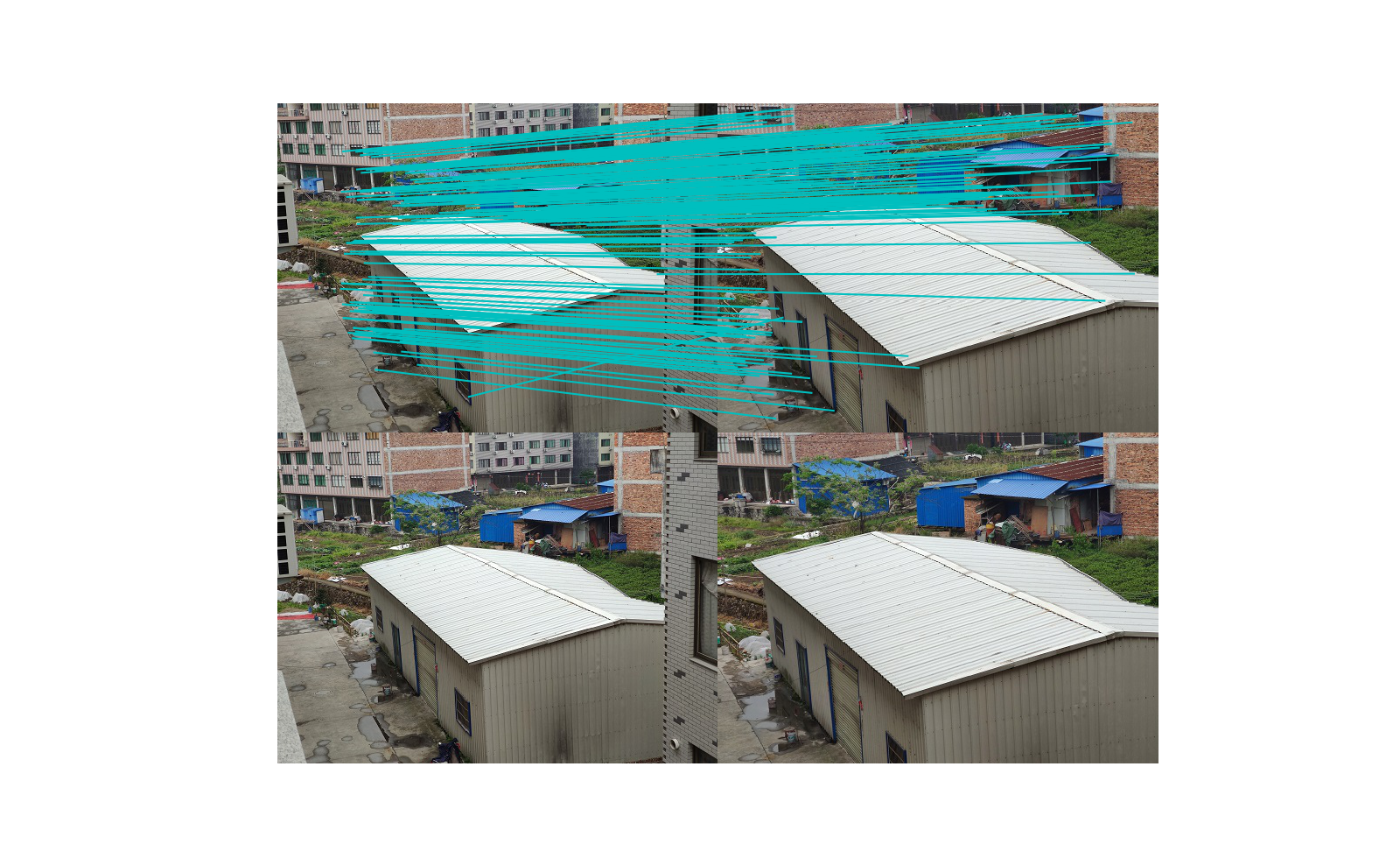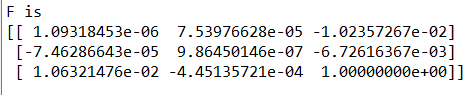#### 3.2画出极点和极线

##### 3.2.1 实验结果
1. 左右变换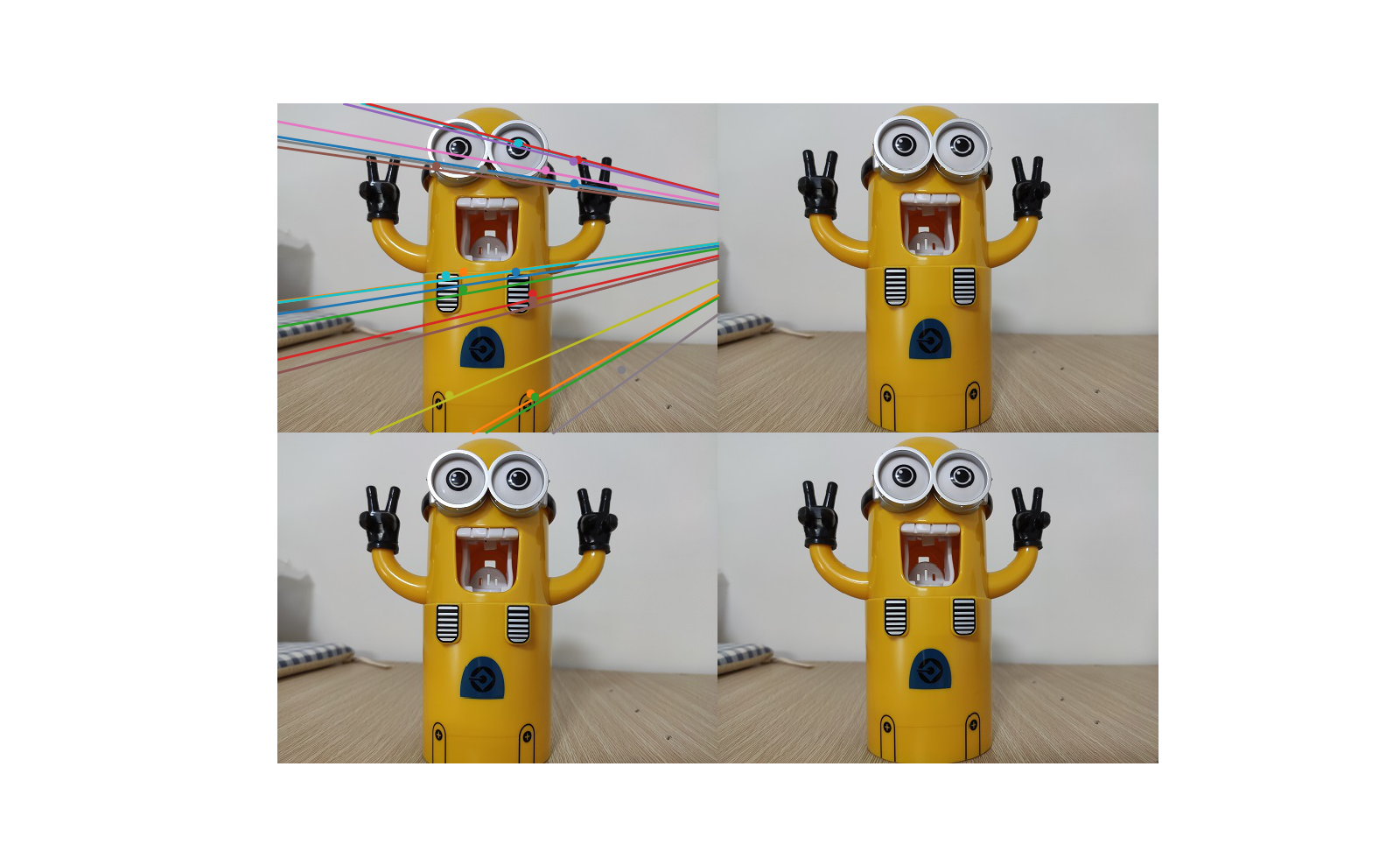1. 平移变换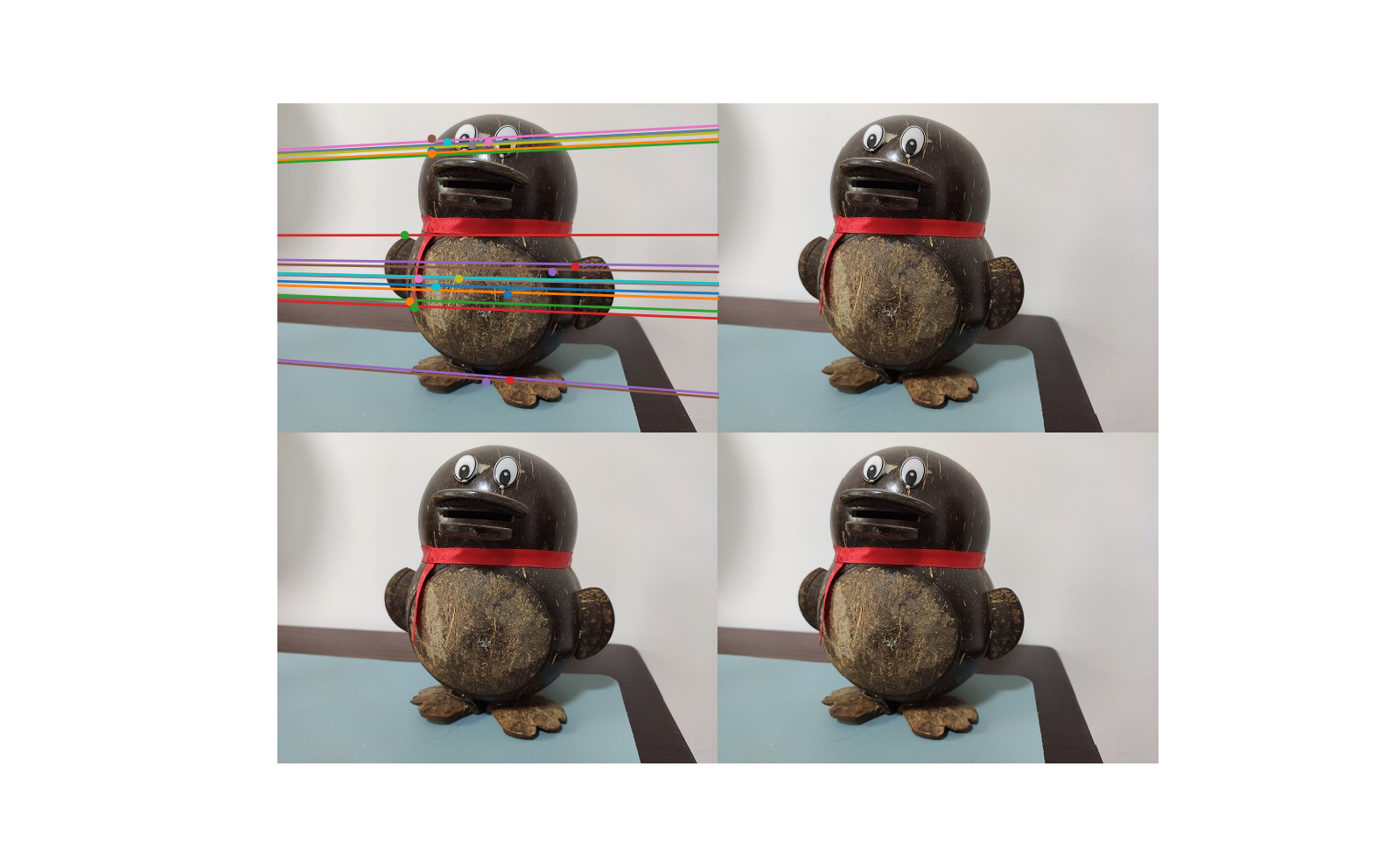1. 前后变换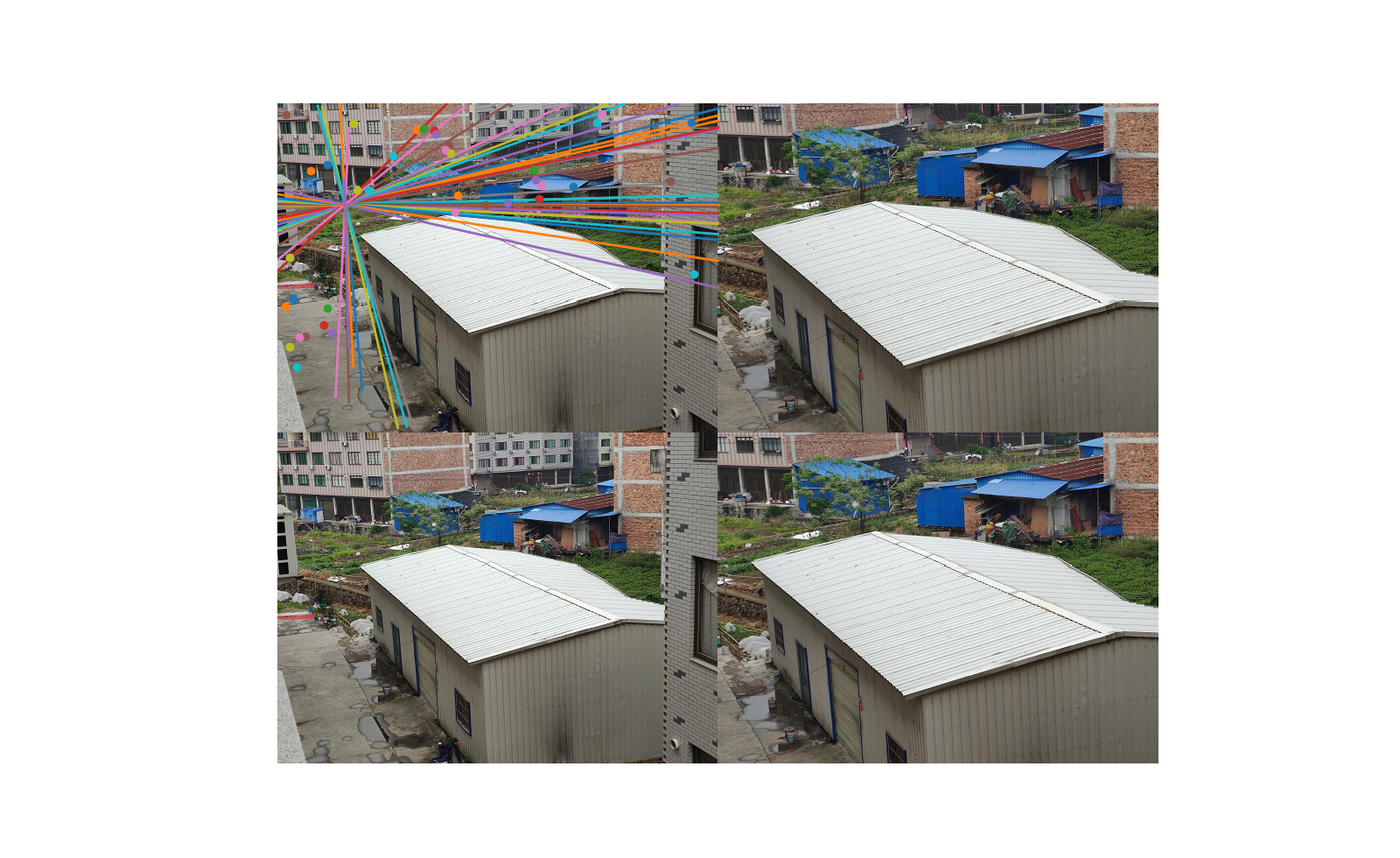##### 3.2.2 分析
1. 在左右不同角度拍摄的图中，极线最终会汇集到一点上，交汇点在图片外，在平行拍摄的两张图上，极线整体上体现的也是平行关系，从前后位拍摄的图中可以看出，极线从四面八方汇集到中心，交汇点在图片中。
2. 以上实验结果显示，极点大部分都会落在极线上，在最后一组上会存在误差。

## 四、问题总结

1. 在处理左右不同角度的图片时，运行出现错误，这是由于图像sift特征匹配的匹配点数过少，可能是因为图像水平落差比较大，经过多次实验发现，拍摄图片时角度选择不能过大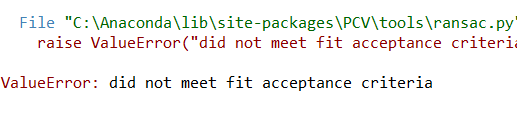展开全文• 在进行极线搜索时，为了方便，将极点通过射影变换转至无穷远点，则图上的极线都变成了平行线，便于搜索

在进行极线搜索时，为了方便，将极点通过射影变换转至无穷远点，则图上的极线都变成了平行线，便于搜索
PAT： 只能在极点位于图像外时使用这种方法，极点在图像内时，极点周围的点也会被变换到无穷远处

## 变换矩阵H的形式

H e = [ 1 , 0 , 0 ] T He=[1,0,0]^T
H H 最少有6个自由度,为了减少畸变，应该使 H H 接近刚体变换, H = G G R G T ∈ R 3 × 3 H=GG_RG_T\in R^{3\times3}
G T = [ 1 0 − O x 0 1 − O y 0 0 1 ] G_T=\begin{bmatrix}1&0&-O_x\\0&1&-O_y\\0&0&1\\\end{bmatrix}
其中 O x , O y O_x,O_y 是图像中心, G T [ O x O y 1 ] = [ 0 0 1 ] G_T\begin{bmatrix}O_x\\O_y\\1\end{bmatrix}=\begin{bmatrix}0\\0\\1\end{bmatrix}

G T G_T 将极点变换到图像坐标系下，再通过刚体变换 G R ∈ S O ( 3 ) G_R\in SO(3) 将极点变换到x轴上, G R G_R 是以z轴为旋转轴的旋转矩阵

G R G T e = [ x e , 0 , 1 ] T G_RG_Te=[x_e,0,1]^T
要使 G [ x e , 0 , 1 ] = [ 1 , 0 , 0 ] T G[x_e,0,1]=[1,0,0]^T ,则 G = [ 1 0 0 0 1 0 − 1 x e 0 1 ] G=\begin{bmatrix}1&0&0\\0&1&0\\ -\frac{1}{x_e}&0&1\end{bmatrix}

## 已标定相机下的极线校正

A compact algorithm for rectification of stereo pairs
这篇论文是在相机已标定情况下讨论的极线校正，两个相机的投影矩阵 P P 都认为已知
P ~ = K [ R ∣ t ] \tilde{P}=K[R|t]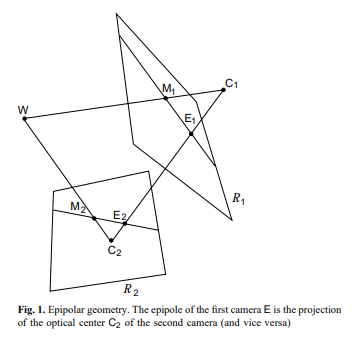论文讲的是如何计算一个矩阵把上面的相机位姿变换成下面图中这种形式，同时使图像的畸变尽量的小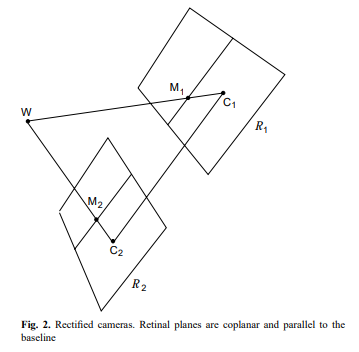可以看到，校正后两个成像平面的x轴和基线平行，也就是两个相机的旋转相同，同时相机在校正的过程中位置 c c 是不能改变的，这样我们就能得到校正后投影矩阵 P P 的大概形式
P n 1 ~ = K R ∣ − R c 1 , P n 2 ~ = K R ∣ − R c 2 \tilde{P_{n1}}=K{R|-Rc_1},\tilde{P_{n2}}=K{R|-Rc_2}
矩阵 R R 可以用他的行向量表示， R = [ r 1 T r 2 T r 3 T ] R=\begin{bmatrix}r_1^T\\r_2^T\\r_3^T\end{bmatrix}
三个行向量分别代表了三个轴在世界坐标系中的单位向量，因为 x x 轴和基线平行，所以 r 1 = ( c 1 − c 2 ) ∣ ∣ c 1 − c 2 ∣ ∣ r_1=\frac{(c_1-c_2)}{||c_1-c_2||} y y 轴要平行于 x x 轴，所以手动设置为 r 2 = k ^ r 1 r_2=\hat{k} r_1 ，其中 k k 是任意单位向量，同时 z z 轴要垂直于 x x 轴和 y y 轴，所以 r 3 = r 1 ^ r 2 r_3=\hat{r_1}r_2 ，这个算法在相机纯平移前后运动时会失效

有了校正后的投影矩阵，我们就可以求解变换矩阵了，这里以左视图为例子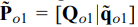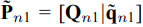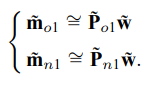W ~ \tilde{W} 是3D点， m ~ \tilde{m} 是对应的投影点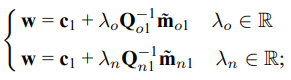可以看到两个图像点变换前后的关系为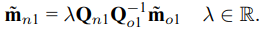变换矩阵为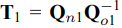论文中一些极线校正的结果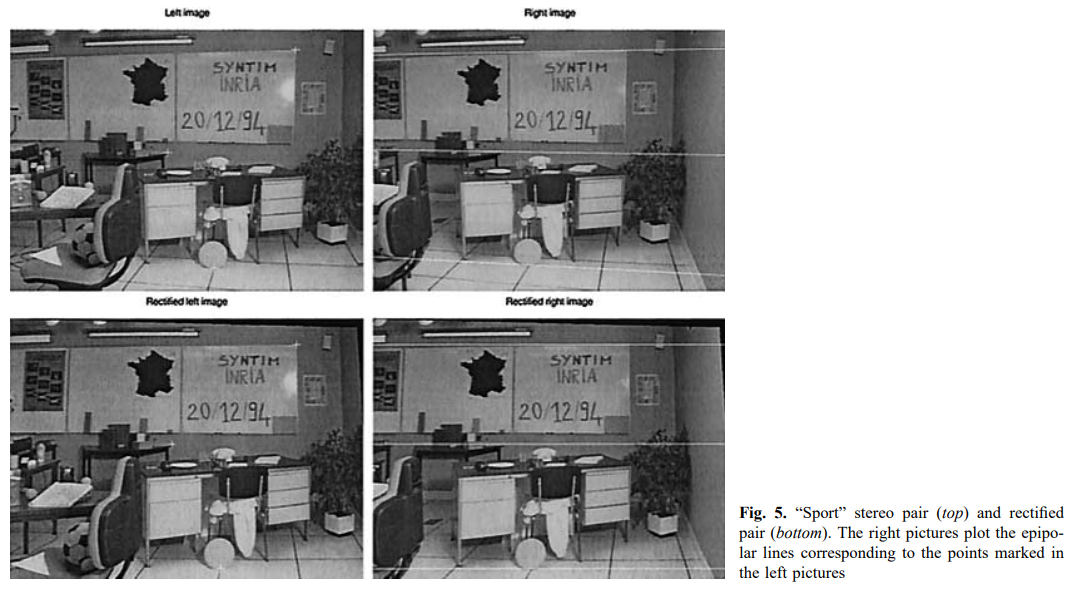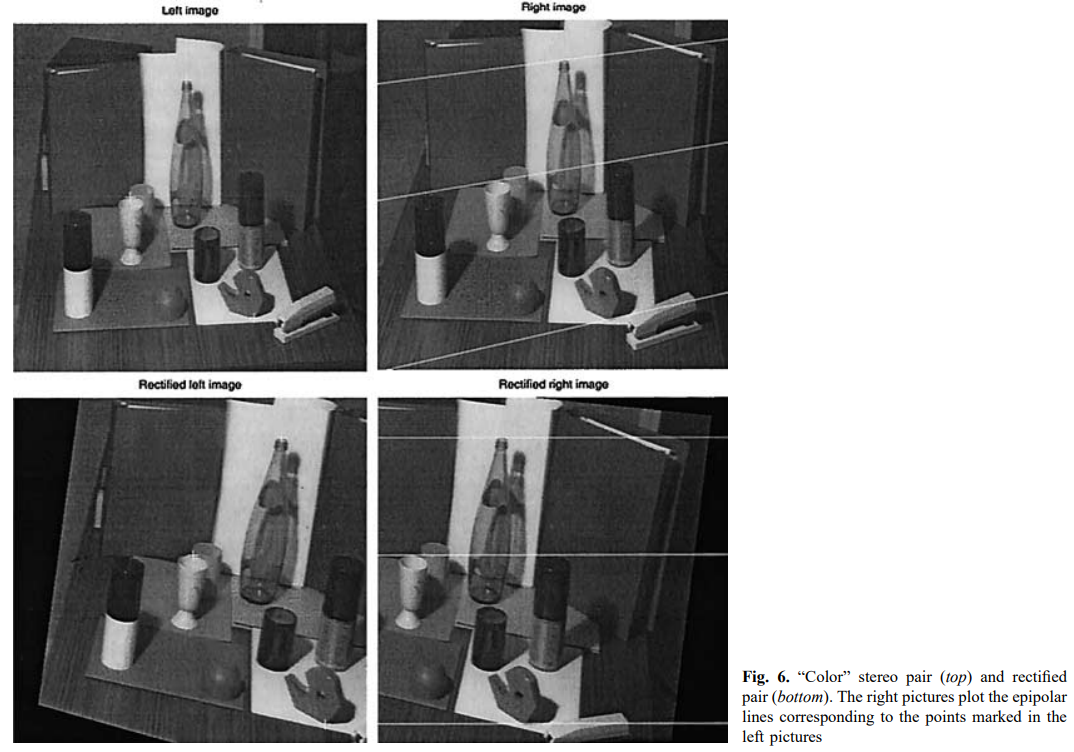## matlab提供的极线校正参考代码

官方文档
matlab中的代码是针对没标定好的相机的，只要两张图之间匹配点大于等于7个并且极点不在图像内就可以完成校正

## 参考文献

Hartley R, Zisserman A. Multiple view geometry in computer vision[M]. Cambridge university press, 2003.

展开全文计算机视觉
• 点击上方“小白学视觉”，选择加"星标"或“置顶”重磅干货，第一时间送达 本文转自：计算机视觉life为什么要做极线校正？三维重建是通过双目立体匹配实现的如图1，通过匹配空...计算机视觉 opencv
• 三角测量和极线校正 ·三角测量 假设两个相机像平面精准位于同一平面上，且行对齐，两个光轴严格平行。 利用三角形关系，我们不难推出Z值：计算机视觉 人工智能
• ## 画出对应的极线

千次阅读 2017-03-29 16:03:52
三维重建 - 摄像机标定和立体匹配中极线约束方法 http://blog.csdn.net/sevencolorfish/article/details/6840624 姿态估计和对极几何 http://blog.sina.com.cn/s/blog_4b9b714a0100c9f3.html画出对应的极线 ...
• 基础矩阵和对极几何一、对极几何1、概念2、外极线、外极点二、基础矩阵1、描述2、实现步骤3、八点法求基础矩阵三、计算基础矩阵1、八点法求基础矩阵2、对比实验 一、对极几何 1、概念 如果有一个场景的两个视图以及...
• 最近在看三维重建的东西，把看到的东西总结一下。一、外极几何已知两个摄像头的光心OO和O′O'，PP为空间中的一点，pp和p′p'是点PP在两个摄像头成的像中的投影。...其中ll是与p′p'相关的外极线，l′l'是与pp
• 双目相机的畸变校正以及平行校正（极线校正）的入门问题总结双目平行校正 相机坐标系
• ## 极线校正原理

千次阅读 热门讨论 2021-06-06 20:06:33
设左相机坐标系为世界坐标系，右相机参为R,T，则左相机坐标系中的点P1，在右相机坐标系中为：P2=RP1+T (1) 极线校正的第一步，是将两个相机各旋转R的一半，令两相机的成像平面平行 首先对R进行罗德里格斯变换，...算法
• 针对传统外极线约束的匹配算法存在误匹配率、漏匹配率较高的问题，提出了基于图像相似几何特征的双目匹配检验和筛选算法。利用外极线几何约束算法获得不共外极线和共外极线的初始匹配点。根据左右图像正确的匹配点...
• 1.极线约束 如上图所示，点P为世界坐标系上一点，p为左图像上一点，p’为有图像上一点，则Ppp’形成一个平面，称为极平面（也称为核平面），左右两条绿线与红线则称为极线；OlO_lOl​与OrO_rOr​则为左右相机的相心...自动驾驶 计算机视觉 图像处理 人工智能
• 通过相机标定的程序获取了两个相机各自的内参矩阵和畸变系数，以及两个相机...平行放置并划线对比显示可见，两幅图像已经达到极线平行： ﻿﻿ ﻿﻿ ﻿﻿ ﻿﻿ ﻿﻿matlab
• 所以可以这样认为，一个极平面就可以在两个视图上找到一对极线，这对极线上的所有像素满足同一个约束公式，而多个极平面就产生了多个极线对，众多的极线对可以涵盖图像上的所有像素点！ 进一步，我们可以将这些极线...算法 人脸识别 编程语言 机器学习 人工智能
• //计算对应点的外极线epilines是一个三元组(a,b,c)，表示点在另一视图中对应的外极线ax+by+c=0; vector < cv : : Vec < float , 3 >> epilines1 , epilines2 ; computeCorrespondEpilines ( pts1 , 1 , ...
• 若要使用自己的图片，请将图片放在\images目录下，参照范例格式命名（0为左，1为右），然后在\data文件夹放入符合格式的_cam（内参矩阵*参矩阵）和_points文件（任意）。 ------------------- 双目立体视觉匹配等...
• 单目：https://blog.csdn.net/zkl99999/article/details/48371877 （注意：opencv 中前五个畸变参数顺序是 [k1, k2, p1, p2, k3]。 matlab 中如果开启了三个径向畸变，[k1, k2, k3] 确实在一起） ...
• 立体视觉入门指南（6）：对级约束与Fusiello法极线校正 原理+代码实战 | 双目视觉中的极线校正 校正目的：对两幅图像的二维匹配搜索变成一维，节省计算量，排除虚假匹配点，成平行视图。 算法流程： 坐标系：...三维重建
• 作为三维重建过程中不可或缺的一步，目前Opencv仅支持实现图像的立体校正，而多数在目标检测情况下需要实现点到点的映射关系，所以尝试借助Opencv的remap实现了任意一组点的极线校正 Opencv极线计算机视觉 opencv 目标检测 三维重建 立体校正
• 极线约束描述的是当同一个点投影到两个不同视角的图像上时，像点、相机光心在投影模型下形成的约束。 如图所示， 两个相机光心连线为O1O2被称为基线，基线与像平面image1和image2的交点e1、e2被称为基点；平面PO1O2...计算机视觉...